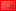##### approximation by fuzzy smoothing cubic splines.
Publication Type
Conference Paper
Authors

One of the most interesting and important techniques in applied mathematics is interpolation and approximation. It can be also shown that basic theory of fuzzy sets and operations with fuzzy numbers can be used, and result very convenient, for solving many analytical chemistry problems: as depth profile comparison or calibration with errors in signals and/or concentrations, for spectra interpretation, or even for automatic qualitative analysis or expert systems with X-ray spectroscopy. In this special context, we introduce in this paper a new fuzzy interpolation method of fuzzy data or functions, specially indicated in all these chemical applications. We will use bicubic splines (cubic splines of two variables) of class C2C2 as linear combinations of a basis constructed by a tensor product of univariate B-splines in each of these variables. First we establish a bicubic interpolation spline method of a given fuzzy data set or a given fuzzy bivariate function. We proof the existence of a unique solution of this problem and we show a convergence result. Finally, we test the effectiveness of this method by some numerical and graphical examples.

Conference
Conference Title
The 7th international conference on approximation methods and numerica modeling in environment and natural resources, (mamern vii 2017)
Conference Country
MoroccoConference Date
May 19, 2017 - May 23, 2017
Conference Sponsor
Journal of mathematics and computer in simulation